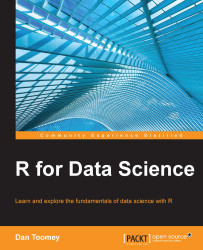•#### R for Data Science#### Overview of this book

R for Data ScienceCreditswww.PacktPub.comPrefaceFree Chapter
Data Mining PatternsData Mining SequencesText MiningData Analysis – Regression AnalysisData Analysis – CorrelationData Analysis – ClusteringData Visualization – R GraphicsData Visualization – PlottingData Visualization – 3DMachine Learning in ActionPredicting Events with Machine LearningSupervised and Unsupervised LearningIndex## Chapter 4. Data Analysis – Regression Analysis

Regression analysis is one of the first tools used when analyzing your dataset. It involves estimating the relationship between variables, and often it will give you an immediate insight into the organization of your data.

In this chapter, we will look at tools available in R for regression analysis:

• Simple regression

• Multiple regression

• Multivariate regression

• Robust regression